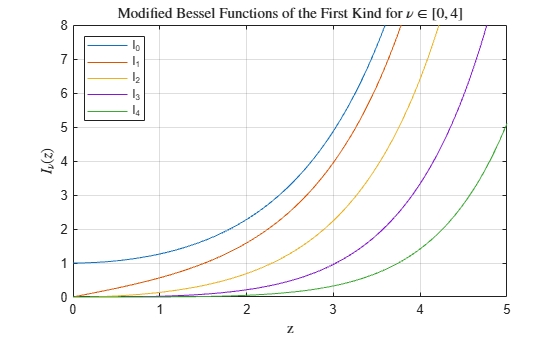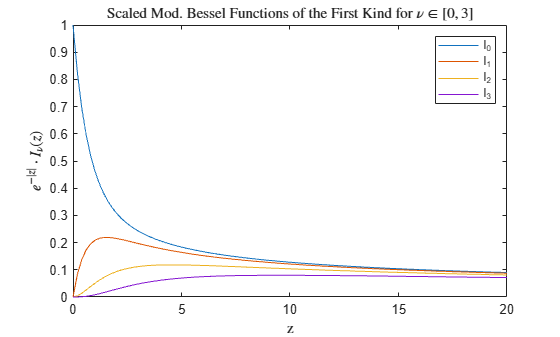# besseli

Modified Bessel function of first kind

## Syntax

I = besseli(nu,Z)
I = besseli(nu,Z,scale)

## Description

example

I = besseli(nu,Z) computes the modified Bessel function of the first kind Iν(z) for each element in array Z.

example

I = besseli(nu,Z,scale) specifies whether to exponentially scale the modified Bessel function of the first kind to avoid overflow or loss of accuracy. If scale is 1, then the output of besseli is scaled by the factor exp(-abs(real(Z))).

## Examples

collapse all

Define the domain.

z = 0:0.01:5;

Calculate the first five modified Bessel functions of the first kind. Each row of I contains the values of one order of the function evaluated at the points in z.

I = zeros(5,501); for nu = 0:4 I(nu+1,:) = besseli(nu,z); end

Plot all of the functions in the same figure.

plot(z,I) axis([0 5 0 8]) grid on legend('I_0','I_1','I_2','I_3','I_4','Location','NorthWest') title('Modified Bessel Functions of the First Kind for $\nu \in [0,4]$','interpreter','latex') xlabel('z','interpreter','latex') ylabel('$I_\nu(z)$','interpreter','latex')Calculate the scaled modified Bessel function of the first kind ${\mathit{I}}_{\nu }\left(\mathit{z}\right)\cdot {\mathit{e}}^{-|\mathrm{Re}\left[\mathit{Z}\right]|}$ for values of $\mathit{z}$ in the interval $\left[0,20\right]$ and for orders $\nu$ between 0 and 3.

z = linspace(0,20); scale = 1; Is = zeros(4,100); for nu = 0:3 Is(nu+1,:) = besseli(nu,z,scale); end

Plot all of the functions in the same figure. For large values of $\mathit{z}$, the scaled functions do not overflow the limits of double precision, extending their range of computability compared to the unscaled functions.

plot(z,Is) legend('I_0','I_1','I_2','I_3') title('Scaled Mod. Bessel Functions of the First Kind for $\nu \in \left[0, 3 \right]$','interpreter','latex') xlabel('z','interpreter','latex') ylabel('$e^{-|{z}|} \cdot I_\nu(z)$','interpreter','latex')## Input Arguments

collapse all

Equation order, specified as a scalar, vector, matrix, or multidimensional array. nu is a real number that specifies the order of the modified Bessel function of the first kind. nu and Z must be the same size, or one of them can be scalar.

Example: besseli(3,Z)

Data Types: single | double

Functional domain, specified as a scalar, vector, matrix, or multidimensional array. besseli is real-valued where Z is positive. nu and Z must be the same size, or one of them can be scalar.

Example: besseli(nu,[1-1i 1+0i 1+1i])

Data Types: single | double
Complex Number Support: Yes

Toggle to scale function, specified as one of these values:

• 0 (default) — No scaling

• 1 — Scale the output of besseli by exp(-abs(real(Z)))

The magnitude of besseli grows rapidly as the value of abs(real(Z)) increases, so exponentially scaling the output is useful for large values of abs(real(Z)), where the results otherwise quickly lose accuracy or overflow the limits of double precision.

Example: besseli(nu,Z,1)

collapse all

### Modified Bessel Functions

This differential equation, where ν is a real constant, is called the modified Bessel's equation:

${z}^{2}\frac{{d}^{2}y}{d{z}^{2}}+z\frac{dy}{dz}-\left({z}^{2}+{\nu }^{2}\right)y=0.$

Its solutions are known as modified Bessel functions.

The modified Bessel functions of the first kind, denoted Iν(z) and Iν(z), form a fundamental set of solutions of the modified Bessel's equation. Iν(z) is defined by

${I}_{\nu }\left(z\right)={\left(\frac{z}{2}\right)}^{\nu }\sum _{\left(k=0\right)}^{\infty }\frac{{\left(\frac{{z}^{2}}{4}\right)}^{k}}{k!\Gamma \left(\nu +k+1\right)}.$

The modified Bessel functions of the second kind, denoted Kν(z), form a second solution independent of Iν(z) given by

${K}_{\nu }\left(z\right)=\left(\frac{\pi }{2}\right)\frac{{I}_{-\nu }\left(z\right)-{I}_{\nu }\left(z\right)}{\mathrm{sin}\left(\nu \pi \right)}\text{\hspace{0.17em}}.$

You can compute the modified Bessel functions of the second kind using besselk.